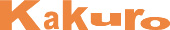﻿ Kakuro
 ВходРегистрация# Kakuro

## Заполните блоки клеток цифрами так, чтобы их сумма совпадала с числом во главе блока

• The number-logic equivalent of crosswords
• Simple rules, very easy to learn
• Requires no language skills
• Wide range of logic and difficulty levels
• Develops logical deduction and reasoning

# Classic Kakuro

Еженедельно - новые головоломки

Each puzzle consists of a blank grid with sum-clues in various places. The object is to fill all empty squares using numbers 1 to 9 so the sum of each horizontal block equals the clue on its left, and the sum of each vertical block equals the clue on its top. In addition, no number may be used in the same block more than once.

Classic Kakuro 7x7
Сложность: очень легкая
Classic Kakuro 8x8
Сложность: очень легкая
Classic Kakuro 10x10
Сложность: очень легкая

# Holey Kakuro

Еженедельно - новые головоломки

Each puzzle consists of a blank grid with sum-clues in various places. The object is to fill all empty squares using numbers 1 to 9 so the sum of each horizontal block equals the clue on its left, and the sum of each vertical block equals the clue on its top. In addition, no number may be used in the same block more than once.

Holey Kakuro 14x14.1
Сложность: легкая
Holey Kakuro 22x16.1
Сложность: средняя

# Round Kakuro

Еженедельно - новые головоломки

Each puzzle consists of a blank grid with sum-clues in various places. The object is to fill all empty squares using numbers 1 to 9 so the sum of each horizontal block equals the clue on its left, and the sum of each vertical block equals the clue on its top. In addition, no number may be used in the same block more than once.

Round Kakuro 10x10.1
Сложность: очень легкая
Round Kakuro 14x14.1
Сложность: легкая

# Multi Kakuro

Еженедельно - новые головоломки

Each puzzle consists of a blank grid with sum-clues in various places. The object is to fill all empty squares using numbers 1 to 9 so the sum of each horizontal block equals the clue on its left, and the sum of each vertical block equals the clue on its top. In addition, no number may be used in the same block more than once.

Multi Kakuro 12x12.1
Сложность: легкая
Multi Kakuro 16x16.3
Сложность: средняя

# Diamond Kakuro

Еженедельно - новые головоломки

Each puzzle consists of a blank grid with sum-clues in various places. The object is to fill all empty squares using numbers 1 to 9 so the sum of each horizontal block equals the clue on its left, and the sum of each vertical block equals the clue on its top. In addition, no number may be used in the same block more than once.

Diamond Kakuro 14x14.1
Сложность: очень легкая
Diamond Kakuro 22x16.1
Сложность: средняя

# Heart Kakuro

Еженедельно - новые головоломки

Each puzzle consists of a blank grid with sum-clues in various places. The object is to fill all empty squares using numbers 1 to 9 so the sum of each horizontal block equals the clue on its left, and the sum of each vertical block equals the clue on its top. In addition, no number may be used in the same block more than once.

Heart Kakuro 12x12.1
Сложность: легкая
Heart Kakuro 14x14.1
Сложность: легкая